• +91 9971497814
• info@interviewmaterial.com

# Chapter 7 Permutations and Combinations Ex-7.3 Interview Questions Answers

### Related Subjects

Question 1 : How many 3-digit numbers can be formed byusing the digits 1 to 9 if no digit is repeated?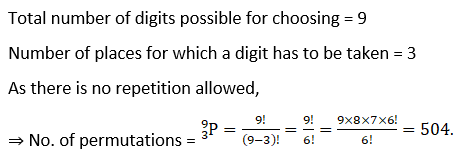Question 2 : How many 4-digit numbers are there with no digit repeated?

To find four digit number (digits does not repeat)
Now we will have 4 places where 4 digits are to be put.
So, at thousand’s place = There are 9 ways as 0 cannot be at thousand’s place = 9 ways
At hundredth’s place = There are 9 digits to be filled as 1 digit is already taken = 9 ways
At ten’s place = There are now 8 digits to be filled as 2 digits are already taken = 8 ways
At unit’s place = There are 7 digits that can be filled = 7 ways
Total Number of ways to fill the four places = 9 × 9 × 8 × 7 = 4536 ways.
So a total of 4536 four digit numbers can be there with no digits repeated.

Question 3 : How many 3-digit even numbers can be made using the digits 1, 2, 3, 4, 6, 7, if no digit is repeated?

Even number means that last digit should be even,
Number of possible digits at one’s place = 3 (2, 4 and 6)
⇒ Number of permutations=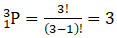One of digit is taken at one’s place, Number of possible digits available = 5
⇒ Number of permutations=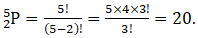Therefore, total number of permutations =3 × 20=60.

Question 4 : Find the number of 4-digit numbers that can be formed using the digits 1, 2, 3, 4, 5 if no digit is repeated. How many of these will be even?

Total number of digits possible for choosing = 5
Number of places for which a digit has to be taken = 4
As there is no repetition allowed,
⇒ Number of permutations =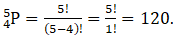The number will be even when 2 and 4 are at one’s place.
The possibility of (2, 4) at one’s place = 2/5 = 0.4
Total number of even number = 120 × 0.4 = 48.

Question 5 : From a committee of 8 persons, in how many ways can we choose a chairman and a vice chairman assuming one person cannot hold more than one position?

Total number of people in committee = 8
Number of positions to be filled = 2
⇒ Number of permutations =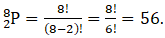Question 6 : Find n if n-1P3nP3 =1: 9.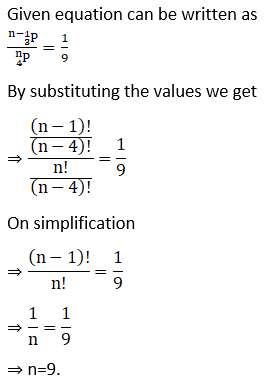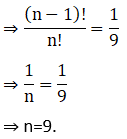Question 7 :

Find r if

(i)5Pr = 26Pr-1

(ii) 5Pr = 6Pr-1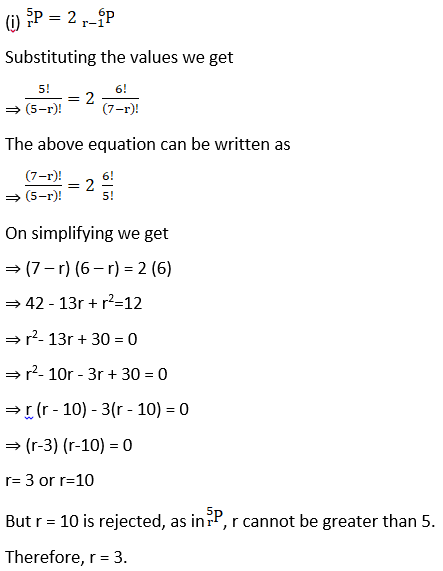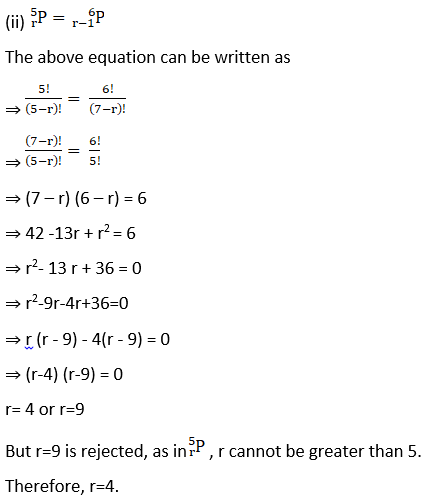Question 8 : How many words, with or without meaning, can be formed using all the letters of the word EQUATION, using each letter exactly once?

Total number of different letters in EQUATION = 8
Number of letters to be used to form a word = 8
⇒ Number of permutations =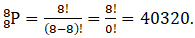Question 9 :
How many words, with or without meaning can be made from the letters of the word MONDAY, assuming that no letter is repeated, if.
(i) 4 letters are used at a time,
(ii) All letters are used at a time,
(iii) all letters are used but first letter is a vowel?

(i) Number of letters to be used =4
⇒ Number of permutations =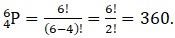(ii) Number of letters to be used = 6
⇒ Number of permutations =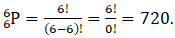(iii) Number of vowels in MONDAY = 2 (O and A)
⇒ Number of permutations in vowel =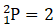Now, remaining places = 5
Remaining letters to be used =5
⇒ Number of permutations =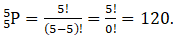Therefore, total number of permutations = 2 × 120 =240.

Question 10 : In how many of the distinct permutations of the letters in MISSISSIPPI do the four I’s not come together?

Total number ofletters in MISSISSIPPI =11

Letter Number ofoccurrence

 M 1 I 4 S 4 P 2
Number ofpermutations =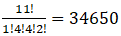We take that 4 I’s come together, and they are treated as 1 letter,
∴ Total number of letters=11 – 4 + 1 = 8
⇒ Number of permutations =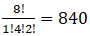Therefore, total number of permutations where four I’s don’t come together = 34650-840=33810

Todays Deals### Chapter 7 Permutations and Combinations Ex-7.3 Contributorskrishan

Name:
Email:

# Latest News# 9000 interview questions in different categories